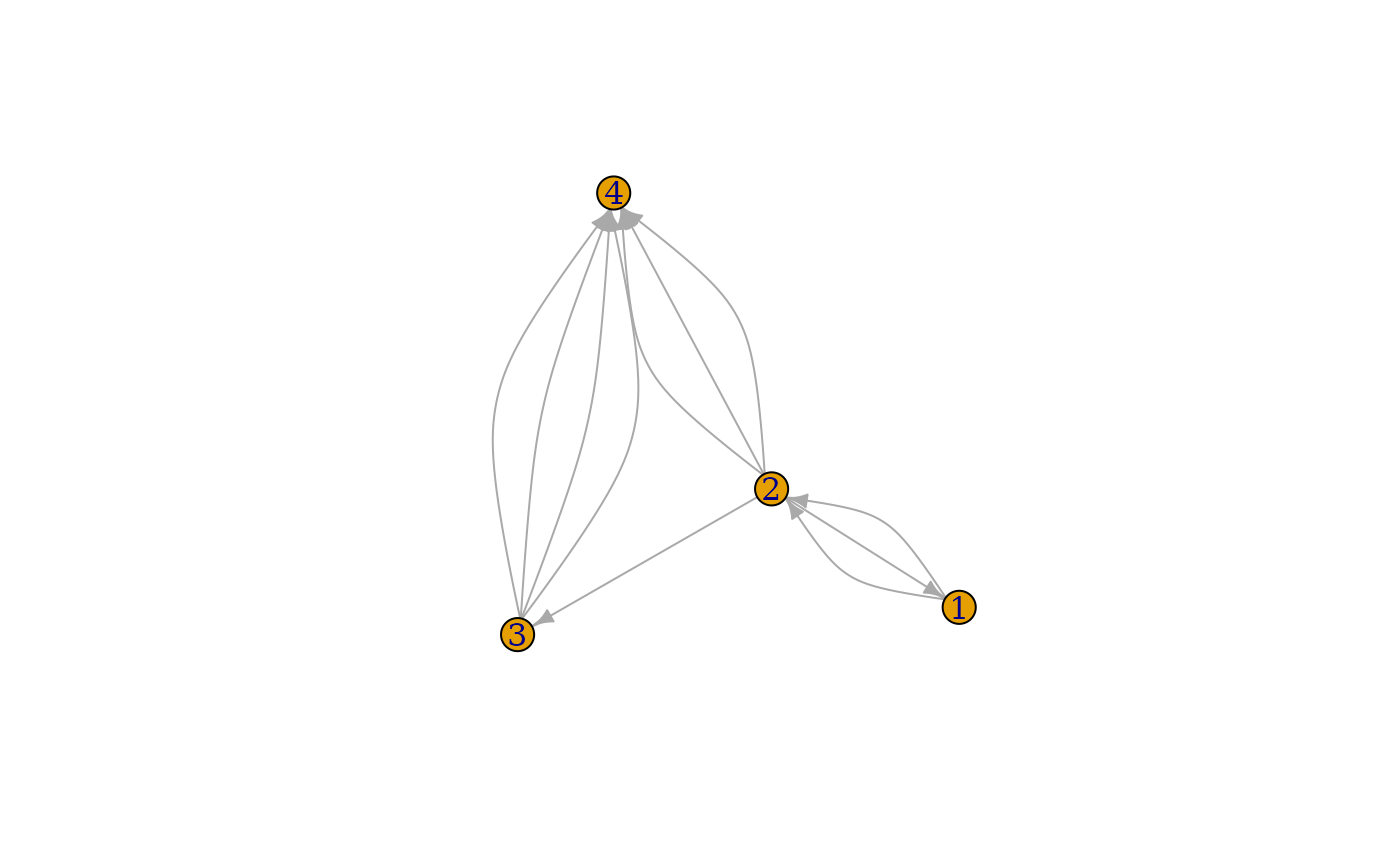If graphs have multiple edges, then drawing them as straight lines does not show them when plotting the graphs; they will be on top of each other. One solution is to bend the edges, with diffenent curvature, so that all of them are visible.

## Usage

curve_multiple(graph, start = 0.5)

## Arguments

graph

The input graph.

start

The curvature at the two extreme edges. All edges will have a curvature between -start and start, spaced equally.

## Value

A numeric vector, its length is the number of edges in the graph.

## Details

curve_multiple() calculates the optimal edge.curved vector for plotting a graph with multiple edges, so that all edges are visible.

igraph.plotting for all plotting parameters, plot.igraph(), tkplot() and rglplot() for plotting functions.

## Author

Gabor Csardi csardi.gabor@gmail.com

## Examples


g <- make_graph(c(
0, 1, 1, 0, 1, 2, 1, 3, 1, 3, 1, 3,
2, 3, 2, 3, 2, 3, 2, 3, 0, 1
) + 1)

curve_multiple(g)
#>   -0.5000000  0.0000000  0.0000000 -0.5000000  0.0000000  0.5000000
#>   -0.5000000 -0.1666667  0.1666667  0.5000000  0.5000000

set.seed(42)
plot(g)